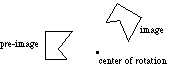index: click on a letter A B C D E F G H I J K L M N O P Q R S T U V W X Y Z A to Z index index: subject areas numbers & symbols sets, logic, proofs geometry algebra trigonometry advanced algebra & pre-calculus calculus advanced topics probability & statistics real world applications multimedia entrieswww.mathwords.com about mathwords website feedback

 Rotation A transformation in which a plane figure turns around a fixed center point. In other words, one point on the plane, the center of rotation, is fixed and everything else on the plane rotates about that point by a given angle.See also